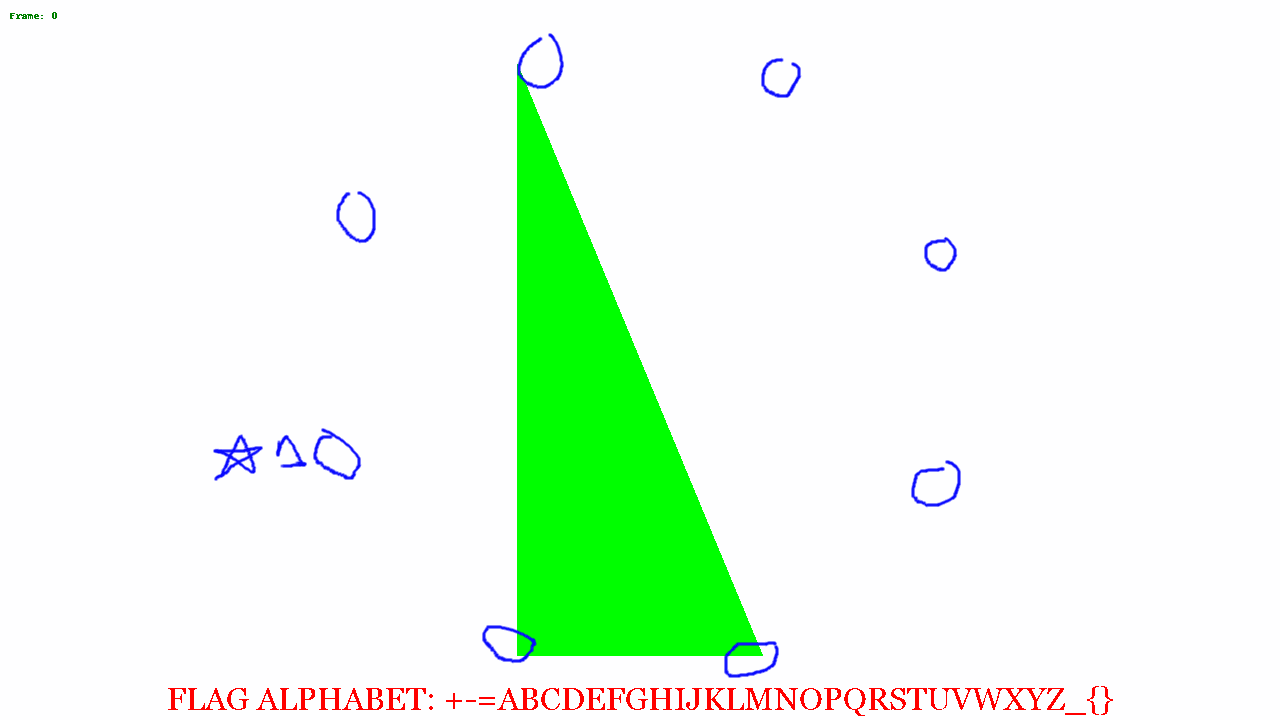# amccormack.net

Things I've learned and suspect I'll forget.

# The Redacted-Puzzle challenge

The challenge prompt says "Everything you need is in this file." and provides a gif.

The gif appears all black so I opened it up in a Jupyter notebook with the Pillow library.I first looked at the number of frames and if the image was animated:

```from PIL import Image
im = Image.open('redacted-puzzle.gif')
print im.is_animated, im.n_frames
```
```True 35
```

To get a quick idea of the variety between the frames, I hashed each individual frame:

```import hashlib
ba = []
for frame in range(im.n_frames):
im.seek(frame)
ba.append(im.tobytes())
print hashlib.md5(ba[-1]).hexdigest(), len(ba[-1]), hashlib.md5(''.join(chr(x) for x in im.getpalette())).hexdigest()
```
```e176bcf3e8b281e91836b1cb2d430405 921600 60a44dc3832c9d6881f7d93298eeb341
acaac3e8a8dbe0531c8dd20146eb66af 921600 60a44dc3832c9d6881f7d93298eeb341
052381a58aacc14ac056f08eafcc13af 921600 60a44dc3832c9d6881f7d93298eeb341
d10c97718cd77ebef4df51b686ca5515 921600 60a44dc3832c9d6881f7d93298eeb341
....
```

This told me that all the frames where the same size, and all were different.

Taking a look at the frames, I realized they were in palette mode, and therefore each byte mapped to a pixel in the image, and the color was determined by the palette.

I converted one of the frames to from pixel to RGBA, and looked at the colors, which are all black or very close to it.

```m = Image.frombytes('P', (1280,720), ba).convert(mode='RGBA')
m.getcolors()
```
```[(6090, (1, 1, 1, 255)), (73112, (2, 2, 2, 255)), (842398, (0, 0, 0, 255))]
```

I put some code together to change the colors and recreate the gif frame by frame. From this we can see various shapes moving around the image.

```import numpy as np
from PIL import ImageFont, ImageDraw
new_colors = []
for i, b in enumerate(ba):
m = Image.frombytes('P', (1280,720), b).convert(mode='RGBA')
data = np.array(m)
r,g,b,a = data.T
white = (r == 0 ) & (b == 0) & (g == 0)
red = (r == 1 ) & (b == 1) & (g == 1)
green = (r == 2) & (b == 2) & (g == 2)
data[..., :-1][white.T] = (255,255,255)
data[..., :-1][red.T] = (255,0,0)
data[..., :-1][green.T] = (0,255,0)
im3 = Image.fromarray(data)
draw = ImageDraw.Draw(im3)
draw.text((10,10), 'Frame: {0}'.format(i), fill='green')
new_colors.append(im3)
new_colors.save('new_redacted.gif', save_all=True, append_images=new_colors[1:], duration=500, loop=0)
```I blended a few of the images together, and saw a distinct hexagonal shape.At this point I wasn't quite sure what to do next. Looking at the alphabet, I noticed there were 32 characters, and I was thinking about base32 encoding. From the octagon, I figured it'd be possible to represent a byte, where each corner of the octagon was a bit, and so the image above, if just the black trapezoid is considered in the image above, the bit patten might read 00001111. There are 35 frames, so 35 bytes = 35 * 8 = 280 bytes. 280 can be evenly divided by 5 so if my base32 theory is correct, there could be a 56 character encoded message.

So I opened the above image in GIMP and circled all the corners and saved the just the circles on a transparent background.I put a star where to start from, and then started putting a 0 or 1 based on if the shape touched or got close to the circle.

I saved off my guesses in a file called `numbers.txt`. Even though I picked a consistent spot to record the pattern, I didn't know if the location I chose was the correct first bit location. So I wrote a script which would take all 35 binary groupings, and rotate each one by place. I would then merge all 35 into one string, and use groups of 5 bits to determine a number, which would make the offset into the alphabet shown in the image.

```alpha = '+-=ABCDEFGHIJKLMNOPQRSTUVWXYZ_{}'
def rotate(x, i):
return x[i:] + x[:i]
with open('numbers.txt') as f:
nums = [ x.split(' ') for x in f.read().split('\n') if x]
for rotate_level in range(8):
p = ''.join(rotate(x, rotate_level) for x in nums)
chunks = [ p[i:i+5] for i in range(0,len(p), 5)]
print rotate_level, ''.join(alpha[int(x,2)] for x in chunks)
```
```0 BMJAQBI-G{F=JL-_B-CQP-V=XISQLR{FIW-J-UAHQSQUPA{LZQOYBA{M
1 FXVUBF{=QVNRVZAXF=IEBANBRUIT_GROUP=FALLREMEMBER_WEATHER}
2 OOO{FORCES-GOVERN+T{FE+FGLU_VQGAMBBNDZ_GLXL{HM-YPLEZRM-}
3 AEAJQA+IMH=AANMG+CKJPL+NPWMYQDPD{FG+KWPQ_N_ZT{ATBZMWH{A{
4 DHDWDDFT{NBTC+{Q+HXGDZ-+CT}EDKJJZRP+WQKDYCYGMZEJGV}=UZEZ
5 JNKPLJOJ_BGJF-_E+ORPMV=+IG{{JXNWWKB-ODXKTMT=}VLWQO{CKVMW
6 W+YBZW=VXJPFPAPM-AGB_NJ+TQ_JYS-PP{FA+KZXJ}JS_N_QEEZHWN}Q
7 PCTGWPCNR_B-DEB{==PWW+V-KEXWUHIBCZNT+YOSWXWYW-YELIVSQ-}E
```

Offset 2 looks really close, we see the start of the flag `OOO{` and it looks like real text and not gibberish. But after 16 or so characters it starts to look like gibberish again. But, The end of offset 1 looks really promising.

I double checked my binary codes against the images noticed that the images periodically fell out of sync with my circles, and that I was guessing for many of them.

I used the following to regenerate all of the frames with a -1 degree rotation and then made a new list of binary codes.

```import numpy as np
from PIL import ImageFilter
from IPython import display
overlayed = []
recolored = []
lay = Image.open('layer2.png')
for i in range(len(ba)):
m = Image.frombytes('P', (1280,720), ba[i]).convert(mode='RGBA')
print i, 'Line {0}'.format(i+1)
data = np.array(m)
r,g,b,a = data.T
black = (r == 0 ) & (b == 0) & (g == 0)
b2 = (r == 1 ) & (b == 1) & (g == 1)
data[..., :-1][black.T] = (255,255,255)
data[..., :-1][b2.T] = (255,255,255)
im3 = Image.fromarray(data)
im3 = im3.rotate(-i)
recolored.append(im3.copy())
im3.alpha_composite(lay)
overlayed.append(im3)
display.display(im3)
```

That produced the following with rotation level 2 putting out the flag:

```0 BMJAQBI-G{F=JL-_B-CWV-V=KEXWUHIBCZNT+YOSWXWYW-YELIVSQ-}E
1 FXVUBF{=QVNRVZAXF=IAPANBXISQLR{FIW-J-UAHQSQUPA{LZQOYBA{M
2 OOO{FORCES-GOVERN+TUBE+FRUIT_GROUP=FALLREMEMBER_WEATHER}
3 AEAJQA+IMH=AANMG+CKLFL+NGLU_VQGAMBBNDZ_GLXL{HM-YPLEZRM-}
4 DHDWDDFT{NBTC+{Q+HXJPZ-+PWMYQDPD{FG+KWPQ_N_ZT{ATBZMWH{A{
5 JNKPLJOJ_BGJF-_E+ORWEV=+CT}EDKJJZRP+WQKDYCYGMZEJGV}=UZEZ
6 W+YBZW=VXJPFPAPM-AG=MNJ+IG{{JXNWWKB-ODXKTMT=}VLWQO{CKVMW
7 PCTGWPCNR_B-DEB{==PR_+V-TQ_JYS-PP{FA+KZXJ}JS_N_QEEZHWN}Q
```

published on 2019-05-12 by alex